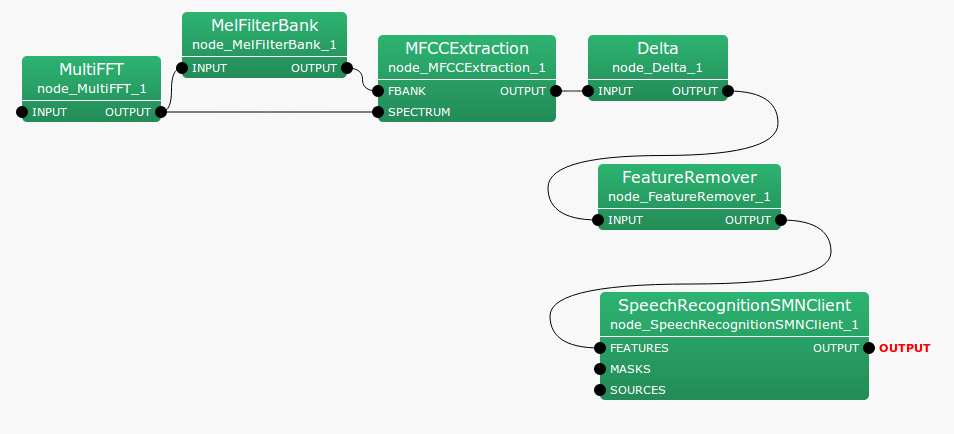## 6.4.4 MFCCExtraction

### 6.4.4.1 Outline of the node

This node acquires mel-cepstrum coefficients (MFCC), which are a type of acoustic feature. It generates acoustic feature vectors consisting of mel-cepstrum coefficients and logarithmic spectrum power as elements.

### 6.4.4.2 Necessary file

No files are required.

### 6.4.4.3 Usage

When to use

This node is used to generate an acoustic feature with mel-cepstrum coefficients as elements and acoustic feature vectors. For example, acoustic feature vectors are input to the speech recognition node to identify phonemes and speakers.

Typical connectionFigure 6.82: Typical connection example of MFCCExtraction

### 6.4.4.4 Input-output and property of the node

Table 6.75: Parameter list of MFCCExtraction
 Parameter name Type Default value Unit Description FBANK_COUNT 24 The number of filter banks for input spectrum NUM_CEPS 12 The number of cepstral coefficients for liftering USE_POWER false Select whether or not to include logarithmic power in features

Input

FBANK

: Map<int, ObjectRef>  type. A pair of the sound source ID and the vector consisting of sound source output energy of the filter bank as Vector<float>  type data.

SPECTRUM

: Map<int, ObjectRef>  type. A pair of the sound source ID and the vector consisting of complex spectra as Vector<complex<float> >  type data.

Output

OUTPUT

: Map<int, ObjectRef>  type. A pair of the sound source ID and the vector consisting of MFCC and a logarithmic power term as Vector<float>  type data.

Parameter

FBANK_COUNT

: int  type. The number of filter banks for the input spectrum. The default value is 24. Its range is positive integer. The frequency band for 1 bank narrows as the value is raised and acoustic features of high frequency resolution are obtained. Acoustic features are expressed more finely by setting greater FBANK_COUNT. Precise expression is not necessarily optimal for speech recognition and it depends on acoustic environment of the utterances.

NUM_CEP

: int  type. The number of cepstrum coefficients on which to perform liftering. The default value is 12. The range is positive integers. When raising the value, the dimension of acoustic features increases. Acoustic features that express finer spectral changes are obtained.

USE_POWER

: When selecting true, the logarithmic power term is added to acoustic features. When selecting false, it is omitted. It is rare to use the power term for acoustic features though it is assumed that delta logarithmic power is effective for speech recognition. When true is selected, delta logarithmic power is calculated in the posterior half and its result is used as acoustic features.

### 6.4.4.5 Details of the node

This node acquires mel-cepstrum coefficients (MFCC), which are one of the acoustic features, and logarithmic power. It generates acoustic features consisting of mel-cepstrum coefficients and log spectrum power as elements. A filter bank with a triangle window is used for log spectra. Center frequencies of triangle windows are positioned at regular intervals on the mel-scale. The output logarithmic energy of each filter bank is extracted and the Discrete Cosine Transform is performed on it. The coefficient for which liftering is performed on the obtained coefficient is the MFCC. It is premised that output logarithmic energy of each filter bank is input to FBANK of the input unit of this node. The vector input to FBANK at frame time $f$ is expressed as follows.

 $\displaystyle \boldsymbol {x}(f)$ $\displaystyle =$ $\displaystyle [ x(f,0),x(f,1), \cdots , x(f,P-1)]^ T$ (136)

Here, $P$ indicates FBANK_COUNT in the dimension number of the input feature vector. The vector output is a $P+1$ dimensional vector and consists of the mel-cepstrum coefficient and power term. The first to $P$th dimensions are for mel-cepstrum coefficients and the dimension $P+1$ is for the power term. The output vector of this node is expressed as;

 $\displaystyle \boldsymbol {y}(f)$ $\displaystyle =$ $\displaystyle [y(f,0),y(f,1),\dots ,y(f,P-1), E]^ T$ (137) $\displaystyle y(f,p)$ $\displaystyle =$ $\displaystyle \displaystyle L(p) \cdot \sqrt {\frac{2}{P}} \cdot \sum _{q=0}^{P-1} \Bigl \{ \log (x(q)) \cos \Bigl ( \frac{ \pi (p+1)(q+0.5)}{P} \Bigr ) \Bigr \}$ (138)

Here, $E$ indicates the power term (see later description). The liftering coefficient is expressed as:

 $\displaystyle L(p)$ $\displaystyle =$ $\displaystyle 1.0 + \frac{Q}{2} \sin \Bigl ( \frac{\pi (p+1)}{Q} \Bigr ),$ (139)

Here, $Q=22$. The power term is obtained from the input vector of SPECTRUM part. The input vector is expressed as:

 $\displaystyle \boldsymbol {s}$ $\displaystyle =$ $\displaystyle [s(0),\dots ,s(K-1)]^ T,$ (140)

Here, $K$ indicates FFT length. $K$ is determined by the dimension number of Map  connected to SPECTRUM. The logarithmic power term is expressed as:

 $\displaystyle E$ $\displaystyle =$ $\displaystyle \log \Bigl ( \frac{1}{K} \sum _{k=0}^{K-1} s(k)\Bigr )$ (141)